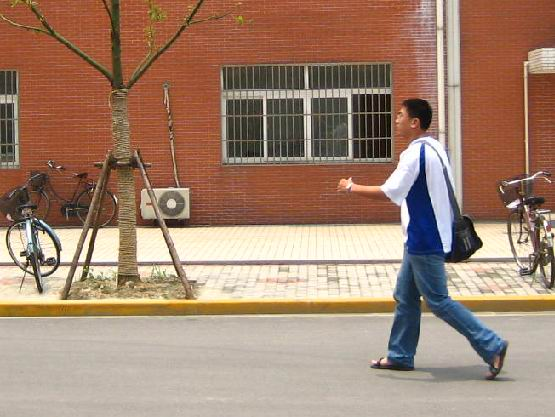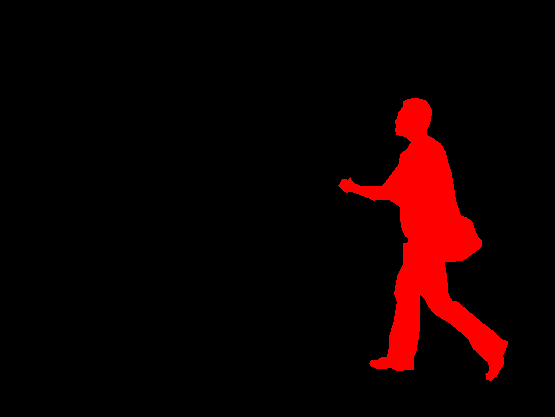In this post, I'll show you how fine-tune Mask-RCNN on a custom dataset. Fine-tune Mask-RCNN is very useful, you can use it to segment specific object and make cool applications.

In a previous post, we've tried fine-tune Mask-RCNN using matterport's implementation. We've seen how to prepare a dataset using VGG Image Annotator (ViA) and how parse json annotations.

This time, we are using PyTorch to train a custom Mask-RCNN. And we are using a different dataset which has mask images (.png files) as . So, we can practice our skills in dealing with different data types. Without any futher ado, let's get into it.

We are using the Pedestrian Detection and Segmentation Dataset from Penn-Fudan Database.

It contains 170 images with 345 instances of pedestrians.

## Customize the Dataset¶

!wget https://www.cis.upenn.edu/~jshi/ped_html/PennFudanPed.zip .
!unzip PennFudanPed.zip


The data is structured as follows

PennFudanPed/
...
PNGImages/
FudanPed00001.png
FudanPed00002.png
FudanPed00003.png
FudanPed00004.png

Let's look at one example from the dataset and it's corresponding segmentation mask.

In :
from PIL import Image
Image.open('PennFudanPed/PNGImages/FudanPed00020.png')

Out:In :
mask = Image.open('PennFudanPed/PedMasks/FudanPed00020_mask.png')
# each mask instance has a different color, from zero to N, where
# N is the number of instances. In order to make visualization easier,
0, 0, 0, # black background
255, 0, 0, # index 1 is red
255, 255, 0, # index 2 is yellow
255, 153, 0, # index 3 is orange
200,200,200, # index 4
])

Out:### Define a Dataset Class to load the data¶

The dataset class should inherit from the standard torch.utils.data.Dataset class, and implement __len__ and __getitem__.

The only specificity that we require is that the dataset __getitem__ should return:

• image: a PIL Image of size (H, W)
• target: a dict containing the following fields
• boxes (FloatTensor[N, 4]): the coordinates of the N bounding boxes in [x0, y0, x1, y1] format, ranging from 0 to W and 0 to H
• labels (Int64Tensor[N]): the label for each bounding box
• image_id (Int64Tensor): an image identifier. It should be unique between all the images in the dataset, and is used during evaluation
• area (Tensor[N]): The area of the bounding box. This is used during evaluation with the COCO metric, to separate the metric scores between small, medium and large boxes.
• iscrowd (UInt8Tensor[N]): instances with iscrowd=True will be ignored during evaluation.
• (optionally) masks (UInt8Tensor[N, H, W]): The segmentation masks for each one of the objects
• (optionally) keypoints (FloatTensor[N, K, 3]): For each one of the N objects, it contains the K keypoints in [x, y, visibility] format, defining the object. visibility=0 means that the keypoint is not visible. Note that for data augmentation, the notion of flipping a keypoint is dependent on the data representation, and you should probably adapt references/detection/transforms.py for your new keypoint representation

If your model returns the above methods, they will make it work for both training and evaluation, and will use the evaluation scripts from pycocotools.

In :
import os
import numpy as np
import torch
import torch.utils.data
from PIL import Image

class PedestrianDataset(torch.utils.data.Dataset):
def __init__(self, root, transforms=None):
self.root = root
self.transforms = transforms
# load all image files, sorting them to
# ensure that they are aligned
self.imgs = list(sorted(os.listdir(os.path.join(root, "PNGImages"))))

def __getitem__(self, idx):
img_path = os.path.join(self.root, "PNGImages", self.imgs[idx])
img = Image.open(img_path).convert("RGB")
# note that we haven't converted the mask to RGB,
# because each color corresponds to a different instance
# with 0 being background

# instances are encoded as different colors
# first id is the background, so remove it
obj_ids = obj_ids[1:]

# split the color-encoded mask into a set

# get bounding box coordinates for each mask
num_objs = len(obj_ids)
boxes = []
for i in range(num_objs):
xmin = np.min(pos)
xmax = np.max(pos)
ymin = np.min(pos)
ymax = np.max(pos)
boxes.append([xmin, ymin, xmax, ymax])

boxes = torch.as_tensor(boxes, dtype=torch.float32)
# there is only one class
labels = torch.ones((num_objs,), dtype=torch.int64)

image_id = torch.tensor([idx])
area = (boxes[:, 3] - boxes[:, 1]) * (boxes[:, 2] - boxes[:, 0])
# suppose all instances are not crowd
iscrowd = torch.zeros((num_objs,), dtype=torch.int64)

target = {}
target["boxes"] = boxes
target["labels"] = labels
target["image_id"] = image_id
target["area"] = area
target["iscrowd"] = iscrowd

if self.transforms is not None:
img, target = self.transforms(img, target)

return img, target

def __len__(self):
return len(self.imgs)


## Define Model Architecture¶

As we want to fine-tune Mask-RCNN, we need to modify its pre-trained head with a new one. For Mask-RCNN, because it has an object-detecor (box_predictor) and a mask_predictor. So, we need to modify both of them to adapt to our dataset.

In :
import torchvision
from torchvision.models.detection.faster_rcnn import FastRCNNPredictor

def build_model(num_classes):
# load an instance segmentation model pre-trained on COCO

# get the number of input features for the classifier
# replace the pre-trained head with a new one

# Stop here if you are fine-tunning Faster-RCNN

# now get the number of input features for the mask classifier
hidden_layer = 256
# and replace the mask predictor with a new one
hidden_layer,
num_classes)

return model


## Training the Model¶

For training process, we will use some helper function from PyTorch Github Repo. They are located in references/detection/ we will use references/detection/engine.py, references/detection/utils.py and references/detection/transforms.py.

!git clone https://github.com/pytorch/vision.git
%cd vision
!git checkout v0.3.0

!cp references/detection/utils.py ../
!cp references/detection/transforms.py ../
!cp references/detection/coco_eval.py ../
!cp references/detection/engine.py ../
!cp references/detection/coco_utils.py ../


In :
from engine import train_one_epoch, evaluate
import utils
import transforms as T

def get_transform(train):
transforms = []
# converts the image, a PIL image, into a PyTorch Tensor
transforms.append(T.ToTensor())
if train:
# during training, randomly flip the training images
# and ground-truth for data augmentation
transforms.append(T.RandomHorizontalFlip(0.5))
return T.Compose(transforms)

# use our dataset and defined transformations
dataset = PedestrianDataset('PennFudanPed', get_transform(train=True))
dataset_test = PedestrianDataset('PennFudanPed', get_transform(train=False))

# split the dataset in train and test set
torch.manual_seed(1)
indices = torch.randperm(len(dataset)).tolist()
dataset = torch.utils.data.Subset(dataset, indices[:-50])
dataset_test = torch.utils.data.Subset(dataset_test, indices[-50:])

# define training and validation data loaders
dataset, batch_size=2, shuffle=True, num_workers=4,
collate_fn=utils.collate_fn)

dataset_test, batch_size=1, shuffle=False, num_workers=4,
collate_fn=utils.collate_fn)


Initialize Model and Optimizer

In :
device = torch.device('cuda') if torch.cuda.is_available() else torch.device('cpu')

# our dataset has two classes only - background and person
num_classes = 2

# get the model using our helper function
model = build_model(num_classes)
# move model to the right device
model.to(device)

# construct an optimizer
params = [p for p in model.parameters() if p.requires_grad]
optimizer = torch.optim.SGD(params, lr=0.005,
momentum=0.9, weight_decay=0.0005)

# and a learning rate scheduler which decreases the learning rate by
# 10x every 3 epochs
lr_scheduler = torch.optim.lr_scheduler.StepLR(optimizer,
step_size=3,
gamma=0.1)


Start Training

Use the following code block to train the model, we train 10 epochs. This trainig process may take a while.

# number of epochs
num_epochs = 10

for epoch in range(num_epochs):
# train for one epoch, printing every 10 iterations
train_one_epoch(model, optimizer, data_loader, device, epoch, print_freq=10)
# update the learning rate
lr_scheduler.step()
# evaluate on the test dataset


After training 10 epochs, we see the log below showing our model's performance on bounding box prediction and segmentation mask predition.

IoU metric: bbox
Average Precision  (AP) @[ IoU=0.50:0.95 | area=   all | maxDets=100 ] = 0.829
Average Precision  (AP) @[ IoU=0.50      | area=   all | maxDets=100 ] = 0.991
Average Precision  (AP) @[ IoU=0.75      | area=   all | maxDets=100 ] = 0.953
Average Precision  (AP) @[ IoU=0.50:0.95 | area= small | maxDets=100 ] = -1.000
Average Precision  (AP) @[ IoU=0.50:0.95 | area=medium | maxDets=100 ] = 0.518
Average Precision  (AP) @[ IoU=0.50:0.95 | area= large | maxDets=100 ] = 0.840
Average Recall     (AR) @[ IoU=0.50:0.95 | area=   all | maxDets=  1 ] = 0.381
Average Recall     (AR) @[ IoU=0.50:0.95 | area=   all | maxDets= 10 ] = 0.873
Average Recall     (AR) @[ IoU=0.50:0.95 | area=   all | maxDets=100 ] = 0.873
Average Recall     (AR) @[ IoU=0.50:0.95 | area= small | maxDets=100 ] = -1.000
Average Recall     (AR) @[ IoU=0.50:0.95 | area=medium | maxDets=100 ] = 0.787
Average Recall     (AR) @[ IoU=0.50:0.95 | area= large | maxDets=100 ] = 0.879
IoU metric: segm
Average Precision  (AP) @[ IoU=0.50:0.95 | area=   all | maxDets=100 ] = 0.760
Average Precision  (AP) @[ IoU=0.50      | area=   all | maxDets=100 ] = 0.991
Average Precision  (AP) @[ IoU=0.75      | area=   all | maxDets=100 ] = 0.931
Average Precision  (AP) @[ IoU=0.50:0.95 | area= small | maxDets=100 ] = -1.000
Average Precision  (AP) @[ IoU=0.50:0.95 | area=medium | maxDets=100 ] = 0.358
Average Precision  (AP) @[ IoU=0.50:0.95 | area= large | maxDets=100 ] = 0.771
Average Recall     (AR) @[ IoU=0.50:0.95 | area=   all | maxDets=  1 ] = 0.349
Average Recall     (AR) @[ IoU=0.50:0.95 | area=   all | maxDets= 10 ] = 0.806
Average Recall     (AR) @[ IoU=0.50:0.95 | area=   all | maxDets=100 ] = 0.806
Average Recall     (AR) @[ IoU=0.50:0.95 | area= small | maxDets=100 ] = -1.000
Average Recall     (AR) @[ IoU=0.50:0.95 | area=medium | maxDets=100 ] = 0.725
Average Recall     (AR) @[ IoU=0.50:0.95 | area= large | maxDets=100 ] = 0.812

Good. Now, you have a customized Mask-RCNN model, you can save it for future use.

torch.save(model, 'mask-rcnn-pedestrian.pt)


## Inference¶

Now our model is ready for making inference. We need to define a few util functions in order to visualize the results. The code below is well explained by the comments.

In [ ]:
# set to evaluation mode
model.eval()
CLASS_NAMES = ['__background__', 'pedestrian']
device = torch.device('cuda') if torch.cuda.is_available() else torch.device('cpu')
model.to(device)

In :
%matplotlib inline
from PIL import Image
import matplotlib.pyplot as plt
import torch
import torchvision.transforms as T
import torchvision
import numpy as np

import cv2
import random
import warnings
warnings.filterwarnings('ignore')

"""
parameters:
method:
- the masks of each predicted object is given random colour for visualization
"""
colours = [[0, 255, 0],[0, 0, 255],[255, 0, 0],[0, 255, 255],[255, 255, 0],[255, 0, 255],[80, 70, 180],[250, 80, 190],[245, 145, 50],[70, 150, 250],[50, 190, 190]]
coloured_mask = np.stack([r, g, b], axis=2)

def get_prediction(img_path, confidence):
"""
get_prediction
parameters:
- img_path - path of the input image
- confidence - threshold to keep the prediction or not
method:
- Image is obtained from the image path
- the image is converted to image tensor using PyTorch's Transforms
- image is passed through the model to get the predictions
ie: eg. segment of cat is made 1 and rest of the image is made 0

"""
img = Image.open(img_path)
transform = T.Compose([T.ToTensor()])
img = transform(img)

img = img.to(device)
pred = model([img])
pred_score = list(pred['scores'].detach().cpu().numpy())
pred_t = [pred_score.index(x) for x in pred_score if x>confidence][-1]
# print(pred['labels'].numpy().max())
pred_class = [CLASS_NAMES[i] for i in list(pred['labels'].cpu().numpy())]
pred_boxes = [[(i, i), (i, i)] for i in list(pred['boxes'].detach().cpu().numpy())]
pred_boxes = pred_boxes[:pred_t+1]
pred_class = pred_class[:pred_t+1]

def segment_instance(img_path, confidence=0.5, rect_th=2, text_size=2, text_th=2):
"""
segment_instance
parameters:
- img_path - path to input image
- confidence- confidence to keep the prediction or not
- rect_th - rect thickness
- text_size
- text_th - text thickness
method:
- prediction is obtained by get_prediction
- each mask is given random color
- each mask is added to the image in the ration 1:0.8 with opencv
- final output is displayed
"""
masks, boxes, pred_cls = get_prediction(img_path, confidence)
img = cv2.cvtColor(img, cv2.COLOR_BGR2RGB)
cv2.rectangle(img, boxes[i], boxes[i],color=(0, 255, 0), thickness=rect_th)
cv2.putText(img,pred_cls[i], boxes[i], cv2.FONT_HERSHEY_SIMPLEX, text_size, (0,255,0),thickness=text_th)
plt.figure(figsize=(20,30))
plt.imshow(img)
plt.xticks([])
plt.yticks([])
plt.show()


Now we are ready to go. Let's see a few examples from our model's inference.

Example 1

In :
!wget -nv https://storage.needpix.com/rsynced_images/pedestrian-zone-456909_1280.jpg -O pedestrian.jpg
segment_instance('./pedestrian.jpg', confidence=0.7)

2020-06-24 08:24:26 URL:https://storage.needpix.com/rsynced_images/pedestrian-zone-456909_1280.jpg [409534/409534] -> "pedestrian.jpg"